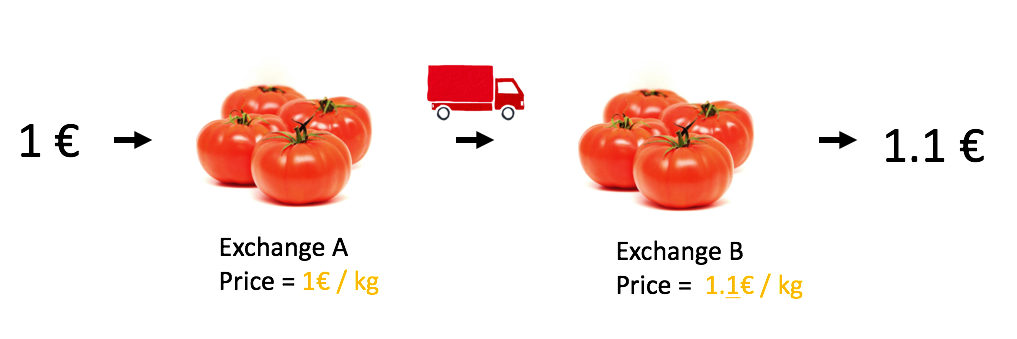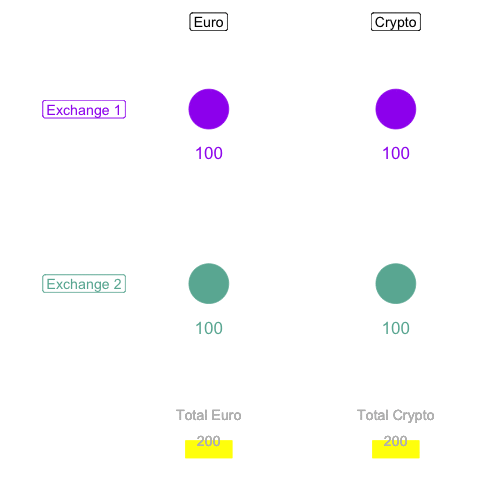HOME

What is arbitrage

What is it? How does it work? What are the potential limits?

“Arbitrage is the practice of taking advantage of a price difference between two or more markets”

# Definition

Crypto currencies are bought and sold on different `exchanges`. Basically it is like a bank, but for crypto. The price is not allways exactly the same on 2 exchanges, as shown here. It is thus possible to buy a crypto where the price is low, and sell it instantly where the price is high to realize a gain without taking any risk.

Consider the following situation as an example:

• step 1 - the price of etherum is 700 euros on Kraken (exchange 1). I take 700 euros and buy 1 Etherum
• step 2 - I send this etherum to Bitstamp (exchange 2)
• step 3 - I sell the etherum on Bitstamp, but the price is different: 750. Thus I now have 750 euros, which is a gain of 50 euros.
• Again

Here is an illustration using tomatoes, buy at 1 euro / kilo, sold at 1.1 euros / kilo. The idea is to do the same, but instantly and using crypto instead of tomatoes.# Limitation

Of course the naive example described above encouters several limit in real life. Here is a list of them:

• `Price differences are rare`: you can read the dedicated page where I quantified these differences
• `Transaction have fees`: each time you buy, sell or transfer crypto, fees apply. In most of the exchanges there is a 0.25% fee to buy and sell
• `Prices evolve quickly`: buy the time you discovered a price discrepancy, prices are already different. This is called Slippage risk. Doing arbitrage without an automatic bot is impossible.
• `Crypto transfer takes time`: you can buy and sell crypto instantly, however it takes time to send it to another exchange or to/from your bank account
• `Concentration risk`: if it is always the same exchange that is more expensive, all your money will ends up in it. You won’t be able to do any new transaction until you rebalance funds.
• `Price volatility`: let’s say you have a efficient arbitrage algorithm that makes 1% a day. If the crypto price drops down when you have some, your loss will probably higher than your gains.

# The plan

Most of the limitations above do not have any workaround. However it is possible to change a bit the arbitrage strategy to avoid transfering funds from one exchange to another. Here is how it works:

• step1 - crypto is cheaper on exchange 1 than on exchange 2. I buy some crypto on it.
• step2 - in the same time, I sell exactly the same amount of crypto on exchange 2.
• step3 - wait for a new price differences in the other side.
• step4 - perform exactly the same think but in the other side.
• Again
``````library(tidyverse)
library(gganimate)
library(tweenr)

# Initial data
init <- data.frame(
x = c(1,4,1,4),
y = c(3,3,1,1),
value = rep(100, 4),
tot = rep(200,4),
tot_crypto = rep(200,4),
arrow1 = rep(0,4),
arrow2 = rep(0,4),
arrow3 = rep(0,4),
arrow4 = rep(0,4)
)

# A function to trade data
gain=1.8
don\$tot <- don\$value + don\$value
don\$tot_crypto <- don\$value + don\$value
don\$arrow1 <- 1
don\$arrow2 <- 0
don\$arrow3 <- 0
don\$arrow4 <- 0
return(don)
}
gain=1.8
don\$tot <- don\$value + don\$value
don\$tot_crypto <- don\$value + don\$value
don\$arrow1 <- 0
don\$arrow2 <- 1
don\$arrow3 <- 0
don\$arrow4 <- 0
return(don)
}
gain=1.8
don\$tot <- don\$value + don\$value
don\$tot_crypto <- don\$value + don\$value
don\$arrow1 <- 0
don\$arrow2 <- 0
don\$arrow3 <- 1
don\$arrow4 <- 0
return(don)
}
gain=1.8
don\$tot <- don\$value + don\$value
don\$tot_crypto <- don\$value + don\$value
don\$arrow1 <- 0
don\$arrow2 <- 0
don\$arrow3 <- 0
don\$arrow4 <- 1
return(don)
}

# Loop to do several transaction
mylist <- list(init, init, init)
tmp <- init
num <- 1
# i is the number of cycle
for(i in 1){
#step1:
num <- num + 1 ; tmp <- trade1(tmp) ; mylist[[num]] <- tmp

#step2
num <- num + 1 ; tmp <- trade1bis(tmp) ; mylist[[num]] <- tmp

#step3
num <- num + 1 ; tmp <- trade2(tmp) ;  mylist[[num]] <- tmp

#step4
num <- num + 1 ; tmp <- trade2bis(tmp) ; mylist[[num]] <- tmp
}
mylist

# Use tweenR
tf <- tween_states(mylist, tweenlength = 0.01, statelength = 0.1, ease = c('cubic-in-out'), nframes = 100)
tf

# Animation
p <- tf   %>% ggplot(aes(x=x, y=y, size=value, frame=.frame)) +
theme_void() +
geom_point( aes(color=paste(x,y))) +
scale_color_manual(values=c("#69b3a2", "purple", "#69b3a2", "purple")) +
scale_size_continuous(range=c(1,30)) +
theme(legend.position="none") +

# Quantity of money
geom_text( aes(label=round(value,0), x=x, y=y-0.5, color=paste(x,y)), size=6) +

# Exchange names
geom_label( x=-1, y=3, label="Exchange 1", color="purple", size=5) +
geom_label( x=-1, y=1, label="Exchange 2", color="#69b3a2", size=5) +

# Arrows: up right / bottom left / bottom right / up left
geom_segment(aes(alpha=arrow1), x=1.8, xend=3.2, y=3, yend=3, size = 1, arrow = arrow(length = unit(0.5, "cm"))) +
geom_segment(aes(alpha=arrow2), x=3.2, xend=1.8, y=1, yend=1, size = 1, arrow = arrow(length = unit(0.5, "cm"))) +
geom_segment(aes(alpha=arrow3), x=1.8, xend=3.2, y=1, yend=1, size = 1, arrow = arrow(length = unit(0.5, "cm"))) +
geom_segment(aes(alpha=arrow4), x=3.2, xend=1.8, y=3, yend=3, size = 1, arrow = arrow(length = unit(0.5, "cm"))) +

# control alpha
scale_alpha_continuous(range=c(0,1)) +

# Barplot money (y=ax + b avec a=(yb-ya)/(xb-xa) et b=y-ax at a given point)
geom_segment(x=1, xend=1, y=-1, aes(yend=(0-(-0.8)/(500-200))*tot-1.33), color="yellow", size=22, alpha=0.7) +
geom_segment(x=4, xend=4, y=-1, aes(yend=(0-(-0.8)/(500-200))*tot_crypto-1.33), color="yellow", size=22, alpha=0.7) +

# Total
geom_text( x=1, y=-.5, label="Total Euro", color="grey", size=5) +
geom_text( x=4, y=-.5, label="Total Crypto", color="grey", size=5) +
geom_text( x=1, y=-.8, aes(label=round(tot,0)), color="grey", size=5) +
geom_text( x=4, y=-.8, aes(label=round(tot_crypto,0)), color="grey", size=5) +

# Money
geom_label( x=1, y=4, label="Euro", color="black", size=5) +
geom_label( x=4, y=4, label="Crypto", color="black", size=5) +

# Limits
xlim(-2,5) +
ylim(-1,4)

gganimate(p, interval = .2, title_frame = F, filename="IMG/arbitrage.gif")``````# Litterature

Several methods of arbitrage exist and I’m far from being the only one trying to do it. Here is a selection of a few really promising project that are worth visiting:

• CryptoArbitrager: use the fact that the evolution of different currencies are strongly correlated. Sometimes a delay is observed, what is an arbitrage opportunity.
• Blackbird: has the advantage of being Market neutral: no loss if the global price of the currency drop down.
• R2
• Peregrine

# Next step

The next step simulates an arbitrage algorithm on a 2 weeks period to assess the potential gain.

A work by Yan Holtz for data-to-viz.com